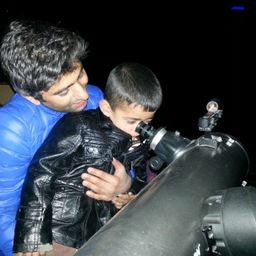Gravitation

### Discussion

You must be signed in to discuss.
##### Top Physics 101 Mechanics Educators##### Marshall S.

University of Washington##### Aspen F.

University of SheffieldLectures

Join Bootcamp

### Video Transcript

well, speed off me on toward earth. We is equal to zero point 990 times the speed of light. This is the speed off me on board arc and distance is also given on distance. D is equal to 4.60 kilometers and 4.60 kilometers is equal to 4.60 Multiply by 10 to the power tree. Meters hard, eh? How long does the moon survive? According to an observer intrest on art well, using s is equal to we multiply by t Well s distance is for 4.60 Multiply by 10 to the power tree speed is given 0.990 times the speed of light Multiply by, uh good know solving for D uh t is equal to 4.60 Multiply retentive Our tree, divided by a cedar 0.990 multiplied by speed of flight and speed of flight is three mil to fire attended or eight meters per second and dividing the two numbers We have 1.55 multiplied by 10 to the power minus five seconds. Okay, now let's do part. Be in part B. We need to find out the latest ick factor. Uh, we know that relativistic factor gamma is equal to one divided by, uh, Square Rudolph one minus we square divided by C square. Let's substitute values. So one divided by, uh, squared Rudolph one minus Well, we is 0.990 times the speed of light hold squared, divided by C square C squared gets canceled with C square and we have one divided by squared Rudolph one minus of a zero point knowing and see the 1901 And therefore they're led. A mystic factor is equal to one divided by, uh, 0.14 107 and dividing this we have, ah, seven point 09 So they're logistic factor is equal to 7.9 Now, let's to part see, we know that it proper time is equal to yeah, down to tea. Divided by the lad Mystic factor Next leg in the values. Well, Delta T identity is 1.55 multiplied by 10 to the power minus five, divided by, uh, gamma and gummy 7.9 And this gives us 2.19 2.19 Multiply by 10 to the power minus six seconds. Now let's to party for, uh, the observer. Traveling with the beyond ah, distance D is equal to we into proper time. Well, we is zero point mine. Mine zero multiplied by three multiply Pretend to devour eight Motive Life dealt it TP which is the proper time, which is 2.19 Multiply by 10 to the power minus six. Yeah, tank LIBOR minus six and therefore D is equal to 650 meters. Elects to Park Hee. All right. Well, the answer to parties. Ah, longer, longer. They're letting the relative speed off the turn Observer with respect to the millions is greater than did off the observer on Earth. This the gamma factor is higher and the lifetime is longer. So the lifetime in life time is longer. Longer. Um#### Topics

Gravitation

##### Top Physics 101 Mechanics Educators##### Marshall S.

University of Washington##### Aspen F.

University of SheffieldLectures

Join Bootcamp